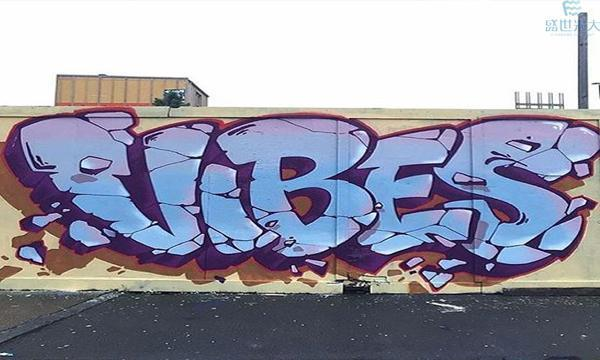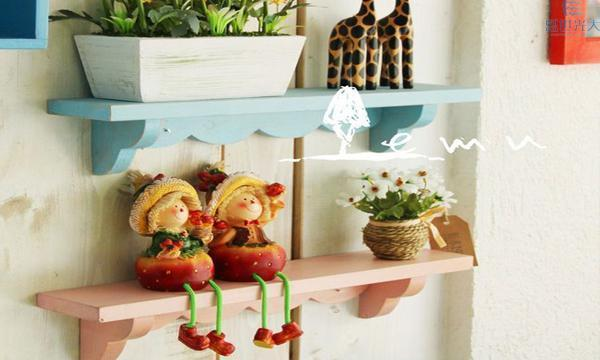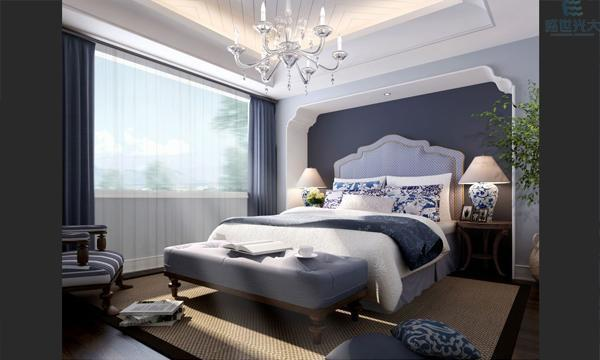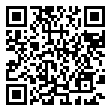|

# 这十项装修风水禁忌你不得不知道!

对于现在大多数人来说，能买到一套价位合适、朝向满意的住房已经相当不容易，所以根本不会过多考虑风水问题，既然风水已成定式，那我们可在装修布局和家居摆设上进行合理的选择，进而有效的改善我们的运势和居住环境。今天成都盛世光大装饰给大家分享10项家装风水格局之禁忌，仅供参考。

NO.1:建筑外观最好不随意更改最好不要在建筑外墙表面装饰一些大型物件，类似大幅图腾、人像等。

从表象来看像是房屋外部长了一坨肉瘤。这种意象不好的外饰装修，在家装风水中应该尽量避免。

解决方法：不要随意改变建筑外观，尽量选择方正、对称等形式。

NO.2:绿色盆栽慎选尽量选择叶面宽阔、寓意美好的绿植。

选择放置家中的绿植，一定要谨慎选择。细长叶片的植物慎选，这种植物放置家中，主人易惹口舌之争。阴性类植物也尽量不选择，类似蕨类，此其长的茂盛，家中易招惹“不干净”的东西。

解决方法：要选宽阔叶面的、或是阳性花卉、盆栽为宜。

NO.3:炉灶的摆放

虽然现在使用炉灶应该比较少，但是炉灶的摆放从古到今都有讲究。摆放位置不可以对着后阳台的门或厨房门。这对常在家中用餐者的健康有损。

解决方法：可以在门上装布帘，作为阻隔。或者将炉灶放置阳台或庭院。

NO.4:阳台不可正对大门出入大门的位置不要正对阳台，这形成了所谓的“穿心”，家中会易漏财，不易聚财之意。

要是住房户型格局是阳台正对大门，可以将窗帘长时间拉上以作为隔断，但这样不利通风。可在客厅阳台处做木质隔断。

解决方法：放置酒柜或玄关柜隔绝大门和阳台。在大门入口处放置鱼缸(命中忌水者不可放鱼缸，可以用屏风取代)或者鞋柜。可设置阳台窗，将阳台阻隔或种植爬藤植物，窗帘长时间拉上也可，不过不利用通风。

NO.5:阳台不可正对厨房

阳台也不可正对厨房。这也是一种“穿心”，会使得家中的团聚功能减弱，丈夫易外遇、老婆会红杏出墙，小孩不爱回家。

解决方法：阳台落地门的窗帘尽量拉上或是作屏风和柜子做阻隔，不让阳台直通厨房即可。做花架种满爬藤植物或放置盆栽，使其内外隔绝。

NO.6:卫生间位置不可在“中宫”上卫生间布局位置在家居风水中，十分重要

要是将房屋的平面九等分，位于正中那一块为家中“中宫”，家居卫生间的位置不能在“中宫”上，即所谓的“厕占中宫”，对居住的家人身体不好以及家庭的财势有所影响。

解决方法：如果装修时已经将坐便器或马桶放置中宫位，试着将洗浴盆和马桶为对调，或者是长期在浴缸中蓄水，便可改善。也可改造水电布局，此法较麻烦。

NO.7:沙发与床不可放在梁的下方要是长沙发已经摆放在横梁下，可以在装修时让天花板的装饰阻断，使看不到横梁

如果沙发与床放在梁的下方，长时间使用沙发和床的人，不仅会有压抑之感，而且会可能招来病痛。

解决方法：长沙发和床避免放在横梁下方，装修的时候将装梁包起来，使其不外漏，即可阻挡之煞气

NO.8:鱼缸不可随意放置并非所有家庭都适合放置鱼缸

虽有聚财之意。但命中忌水者，家中不可放鱼缸，装修入住，居住者五行喜忌须清楚，有特别忌讳者，家中都应避免。

解决方法：查看万年历中的居住者五行喜忌，家中有禁忌者应避免放置犯冲属性物品。

NO.9:遮雨棚的垂檐要做成弧形

阳台遮雨棚的垂檐，不可呈箭形，对常进出阳台者身体不利。

解决方法：不做垂檐，如一定要做垂檐，尽量作弧形而不要做成尖的形状。

NO.10:家中不要随意安放明镜家中明镜不要随意安装，避免破坏室内磁场

现在很多住宅户型较小，为了达到扩宽室内空间的效果，会很喜欢采用安放镜面作为装饰，但这种方式适合与放于客厅，卧房最好不要装大面的镜子，会使得磁场因反射而紊乱。引起居住者神经衰弱、气色不好。

解决方法：如果真的要安装镜面，最好放一面即可，两面会反射。或者安装布帘做遮挡，还有卧室镜面可藏于衣柜。

图片来源网络如侵则删，文章编辑盛世光大运营人员，未经授权禁止转载。

盛世光大装饰作为一站式移动整装平台，我们不仅仅拥有一波强大的工程管理团队，2017年，公司携手家私巨头南方家私，联合打造国内一线“一站式移动装饰”平台，在中国家居产业中已经形成规模化、品牌化、集团化、产业化的绝对领导优势、构建起中国最具竞争力的装饰产业和品牌梯队。公司提出了“智能化、模块化、个性化、金融化、物联化”五化战略指导思想，形成齐发并举的战略体系和格局，计划3年打造一千家超规模整装平台，成为全国龙头装饰企业。 盛世光大拥有近150位高水准，极富创意且行业经验丰富的室内设计人员，近400余名专业施工、管理人员，员工数量超过1500人。超过12000平米材料展厅，拥有一级装饰资质，公司引进洛奢尼工艺，始终以环保家装为基础，用精雕细琢的手工和先进的工艺理念，以及丰富的经验，为社会倾力打造品质环保的绿色空间，为全国各地的客户提供最环保、最创意、最贴心、最专业、最实惠、最高满意度的幸福“家”装体验，位中国家居行业持续贡献力量。 我们最求卓越，不断创新，优化服务，我们只做环保家装！

盛世光大，我们只做环保家装。

`声明：本文由入驻焦点开放平台的作者撰写，除焦点官方账号外，观点仅代表作者本人，不代表焦点立场错误信息举报电话： 400-099-0099，邮箱：jubao@vip.sohu.com，或点此进行意见反馈，或点此进行举报投诉。`A B C D E F G H J K L M N P Q R S T W X Y Z
A - B - C - D - E
• A
• 鞍山
• 安庆
• 安阳
• 安顺
• 安康
• 澳门
• B
• 北京
• 保定
• 包头
• 巴彦淖尔
• 本溪
• 蚌埠
• 亳州
• 滨州
• 北海
• 百色
• 巴中
• 毕节
• 保山
• 宝鸡
• 白银
• 巴州
• C
• 承德
• 沧州
• 长治
• 赤峰
• 朝阳
• 长春
• 常州
• 滁州
• 池州
• 长沙
• 常德
• 郴州
• 潮州
• 崇左
• 重庆
• 成都
• 楚雄
• 昌都
• 慈溪
• 常熟
• D
• 大同
• 大连
• 丹东
• 大庆
• 东营
• 德州
• 东莞
• 德阳
• 达州
• 大理
• 德宏
• 定西
• 儋州
• 东平
• E
• 鄂尔多斯
• 鄂州
• 恩施
F - G - H - I - J
• F
• 抚顺
• 阜新
• 阜阳
• 福州
• 抚州
• 佛山
• 防城港
• G
• 赣州
• 广州
• 桂林
• 贵港
• 广元
• 广安
• 贵阳
• 固原
• H
• 邯郸
• 衡水
• 呼和浩特
• 呼伦贝尔
• 葫芦岛
• 哈尔滨
• 黑河
• 淮安
• 杭州
• 湖州
• 合肥
• 淮南
• 淮北
• 黄山
• 菏泽
• 鹤壁
• 黄石
• 黄冈
• 衡阳
• 怀化
• 惠州
• 河源
• 贺州
• 河池
• 海口
• 红河
• 汉中
• 海东
• 怀来
• I
• J
• 晋中
• 锦州
• 吉林
• 鸡西
• 佳木斯
• 嘉兴
• 金华
• 景德镇
• 九江
• 吉安
• 济南
• 济宁
• 焦作
• 荆门
• 荆州
• 江门
• 揭阳
• 金昌
• 酒泉
• 嘉峪关
K - L - M - N - P
• K
• 开封
• 昆明
• 昆山
• L
• 廊坊
• 临汾
• 辽阳
• 连云港
• 丽水
• 六安
• 龙岩
• 莱芜
• 临沂
• 聊城
• 洛阳
• 漯河
• 娄底
• 柳州
• 来宾
• 泸州
• 乐山
• 六盘水
• 丽江
• 临沧
• 拉萨
• 林芝
• 兰州
• 陇南
• M
• 牡丹江
• 马鞍山
• 茂名
• 梅州
• 绵阳
• 眉山
• N
• 南京
• 南通
• 宁波
• 南平
• 宁德
• 南昌
• 南阳
• 南宁
• 内江
• 南充
• P
• 盘锦
• 莆田
• 平顶山
• 濮阳
• 攀枝花
• 普洱
• 平凉
Q - R - S - T - W
• Q
• 秦皇岛
• 齐齐哈尔
• 衢州
• 泉州
• 青岛
• 清远
• 钦州
• 黔南
• 曲靖
• 庆阳
• R
• 日照
• 日喀则
• S
• 石家庄
• 沈阳
• 双鸭山
• 绥化
• 上海
• 苏州
• 宿迁
• 绍兴
• 宿州
• 三明
• 上饶
• 三门峡
• 商丘
• 十堰
• 随州
• 邵阳
• 韶关
• 深圳
• 汕头
• 汕尾
• 三亚
• 三沙
• 遂宁
• 山南
• 商洛
• 石嘴山
• T
• 天津
• 唐山
• 太原
• 通辽
• 铁岭
• 泰州
• 台州
• 铜陵
• 泰安
• 铜仁
• 铜川
• 天水
• 天门
• W
• 乌海
• 乌兰察布
• 无锡
• 温州
• 芜湖
• 潍坊
• 威海
• 武汉
• 梧州
• 渭南
• 武威
• 吴忠
• 乌鲁木齐
X - Y - Z
• X
• 邢台
• 徐州
• 宣城
• 厦门
• 新乡
• 许昌
• 信阳
• 襄阳
• 孝感
• 咸宁
• 湘潭
• 湘西
• 西双版纳
• 西安
• 咸阳
• 西宁
• 仙桃
• 西昌
• Y
• 运城
• 营口
• 盐城
• 扬州
• 鹰潭
• 宜春
• 烟台
• 宜昌
• 岳阳
• 益阳
• 永州
• 阳江
• 云浮
• 玉林
• 宜宾
• 雅安
• 玉溪
• 延安
• 榆林
• 银川
• Z
• 张家口
• 镇江
• 舟山
• 漳州
• 淄博
• 枣庄
• 郑州
• 周口
• 驻马店
• 株洲
• 张家界
• 珠海
• 湛江
• 肇庆
• 中山
• 自贡
• 资阳
• 遵义
• 昭通
• 张掖
• 中卫

1室1厅1厨1卫1阳台

1
2
3
4
5

0
1
2

1

1

0
1
2
3报名成功，资料已提交审核A B C D E F G H J K L M N P Q R S T W X Y Z
A - B - C - D - E
• A
• 鞍山
• 安庆
• 安阳
• 安顺
• 安康
• 澳门
• B
• 北京
• 保定
• 包头
• 巴彦淖尔
• 本溪
• 蚌埠
• 亳州
• 滨州
• 北海
• 百色
• 巴中
• 毕节
• 保山
• 宝鸡
• 白银
• 巴州
• C
• 承德
• 沧州
• 长治
• 赤峰
• 朝阳
• 长春
• 常州
• 滁州
• 池州
• 长沙
• 常德
• 郴州
• 潮州
• 崇左
• 重庆
• 成都
• 楚雄
• 昌都
• 慈溪
• 常熟
• D
• 大同
• 大连
• 丹东
• 大庆
• 东营
• 德州
• 东莞
• 德阳
• 达州
• 大理
• 德宏
• 定西
• 儋州
• 东平
• E
• 鄂尔多斯
• 鄂州
• 恩施
F - G - H - I - J
• F
• 抚顺
• 阜新
• 阜阳
• 福州
• 抚州
• 佛山
• 防城港
• G
• 赣州
• 广州
• 桂林
• 贵港
• 广元
• 广安
• 贵阳
• 固原
• H
• 邯郸
• 衡水
• 呼和浩特
• 呼伦贝尔
• 葫芦岛
• 哈尔滨
• 黑河
• 淮安
• 杭州
• 湖州
• 合肥
• 淮南
• 淮北
• 黄山
• 菏泽
• 鹤壁
• 黄石
• 黄冈
• 衡阳
• 怀化
• 惠州
• 河源
• 贺州
• 河池
• 海口
• 红河
• 汉中
• 海东
• 怀来
• I
• J
• 晋中
• 锦州
• 吉林
• 鸡西
• 佳木斯
• 嘉兴
• 金华
• 景德镇
• 九江
• 吉安
• 济南
• 济宁
• 焦作
• 荆门
• 荆州
• 江门
• 揭阳
• 金昌
• 酒泉
• 嘉峪关
K - L - M - N - P
• K
• 开封
• 昆明
• 昆山
• L
• 廊坊
• 临汾
• 辽阳
• 连云港
• 丽水
• 六安
• 龙岩
• 莱芜
• 临沂
• 聊城
• 洛阳
• 漯河
• 娄底
• 柳州
• 来宾
• 泸州
• 乐山
• 六盘水
• 丽江
• 临沧
• 拉萨
• 林芝
• 兰州
• 陇南
• M
• 牡丹江
• 马鞍山
• 茂名
• 梅州
• 绵阳
• 眉山
• N
• 南京
• 南通
• 宁波
• 南平
• 宁德
• 南昌
• 南阳
• 南宁
• 内江
• 南充
• P
• 盘锦
• 莆田
• 平顶山
• 濮阳
• 攀枝花
• 普洱
• 平凉
Q - R - S - T - W
• Q
• 秦皇岛
• 齐齐哈尔
• 衢州
• 泉州
• 青岛
• 清远
• 钦州
• 黔南
• 曲靖
• 庆阳
• R
• 日照
• 日喀则
• S
• 石家庄
• 沈阳
• 双鸭山
• 绥化
• 上海
• 苏州
• 宿迁
• 绍兴
• 宿州
• 三明
• 上饶
• 三门峡
• 商丘
• 十堰
• 随州
• 邵阳
• 韶关
• 深圳
• 汕头
• 汕尾
• 三亚
• 三沙
• 遂宁
• 山南
• 商洛
• 石嘴山
• T
• 天津
• 唐山
• 太原
• 通辽
• 铁岭
• 泰州
• 台州
• 铜陵
• 泰安
• 铜仁
• 铜川
• 天水
• 天门
• W
• 乌海
• 乌兰察布
• 无锡
• 温州
• 芜湖
• 潍坊
• 威海
• 武汉
• 梧州
• 渭南
• 武威
• 吴忠
• 乌鲁木齐
X - Y - Z
• X
• 邢台
• 徐州
• 宣城
• 厦门
• 新乡
• 许昌
• 信阳
• 襄阳
• 孝感
• 咸宁
• 湘潭
• 湘西
• 西双版纳
• 西安
• 咸阳
• 西宁
• 仙桃
• 西昌
• Y
• 运城
• 营口
• 盐城
• 扬州
• 鹰潭
• 宜春
• 烟台
• 宜昌
• 岳阳
• 益阳
• 永州
• 阳江
• 云浮
• 玉林
• 宜宾
• 雅安
• 玉溪
• 延安
• 榆林
• 银川
• Z
• 张家口
• 镇江
• 舟山
• 漳州
• 淄博
• 枣庄
• 郑州
• 周口
• 驻马店
• 株洲
• 张家界
• 珠海
• 湛江
• 肇庆
• 中山
• 自贡
• 资阳
• 遵义
• 昭通
• 张掖
• 中卫• 手机• 分享
• 设计
免费设计
• 计算器
装修计算器
• 入驻
合作入驻
• 联系
联系我们
• 置顶
返回顶部